## Correlation Analysis using R and Python

By: Ram on Aug 04, 2020

## Concept Overview

Correlation analysis is used to measure the strength of the association (linear relationship) between two variables and these two variables have to be continuous variables.

One of the first steps in understanding the relationship is to visualize using the Scatter Plot. For example, the below scatter plot shows the relationship between Balance and Spend.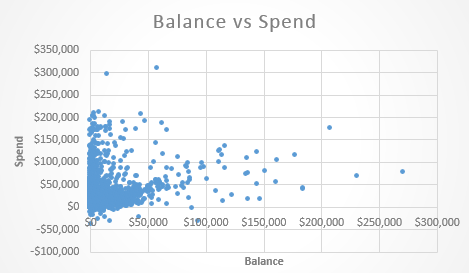The next step is to find a quantitative measure of relationship and one of the statistics used as a measure of relationships is called the Pearson Correlation Coefficient, ρ (rho) or r.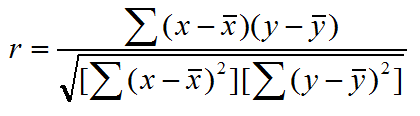Some of the points to be noted related to correlation analysis are mentioned below

• Only concerned with the strength and direction of the relationship and does not imply a causal relationship
• Correlation coefficient ρ (rho) is unit free and range between -1 and 1
• The closer to -1, the stronger the negative linear relationship
• The closer to 1, the stronger the positive linear relationship
• The closer to 0, the weaker the linear relationship

If you wanted detailed steps of Correlation Coefficient Calculations, you could download this

### Scenario

For the credit card portfolio, the business manager may be interested to find a relationship between total spend on a credit card and the average monthly balance. In the data, the last 6 months’ total Spend and average Balances is available for the analysis.

## Correlation Analysis using R

```cardSpendBal=read.csv(file="http://ramgopalprajapat.com/static/files/2020/08/04/card_spend_balance.csv") View(cardSpendBal)```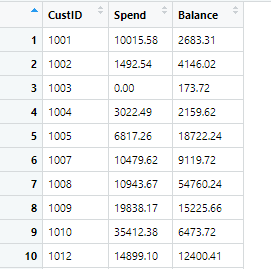### Scatter Plot and a Regression Line

```# Scatter Plot plot(cardSpendBal\$Spend,      cardSpendBal\$Balance,       pch=20,       col='blue',      xlab="Spend",      ylab = "Balance",      main="Relationship Between Spend & Balance")```

```# Add relationship Line abline(lm(cardSpendBal\$Balance~cardSpendBal\$Spend), col="red")```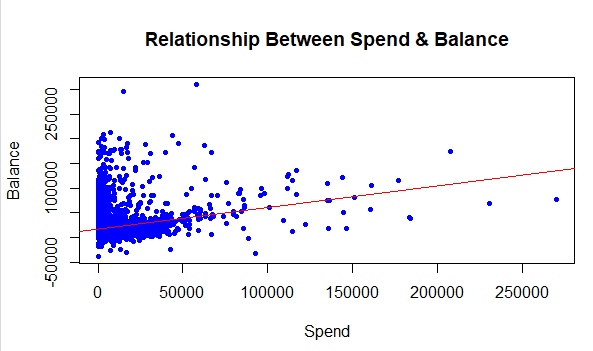### Person Correlation Coefficient

```# Person Correlation cor(cardSpendBal[,2:3],      use="complete.obs",     method="pearson") ```

```# P Value cor.test(cardSpendBal[,2], # X variable/Spend          cardSpendBal[,3], # Y variable/Balance          alternative = c("two.sided"), # Two Tailed Hypothesis          method = c("pearson"), # Person Correlation Method )```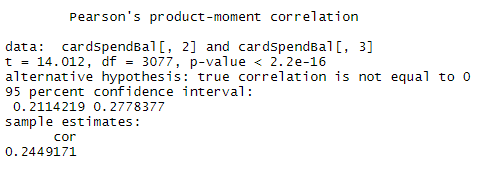### Observations:

There is a positive relationship between spend and balance. The level of relationship based on the Pearson correlation coefficient value of 0.24, we can say that the relationship is NOT very strong (close to 1 indicates a high level of positive relationship).  P-value suggests the current level of relationship is significant - will be valid across samples.

## Correlation Analysis using Python

```import pandas as pd # Read data cardSpendBal=pd.read_csv("http://ramgopalprajapat.com/static/files/2020/08/04/card_spend_balance.csv") # View rows cardSpendBal.head()```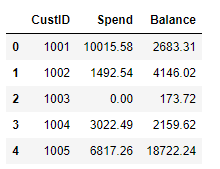### Scatter Plot - Spend vs Balance

```# Scatter Plot  import matplotlib.pyplot as plt plt.scatter(cardSpendBal.loc[:,['Spend']], cardSpendBal.loc[:,['Balance']]) plt.xlabel("Spend") plt.ylabel("Balance") plt.title("Relationship Between Spend & Balance") plt.show()```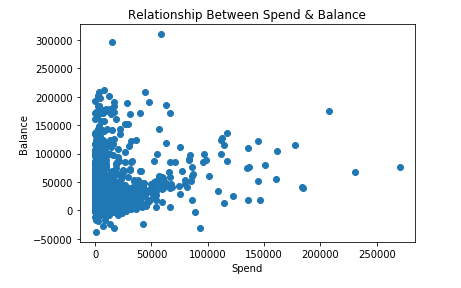### Scatter Plot with Regression Line

```from sklearn.linear_model import LinearRegression # Add Regression Line # y =mx+c - Estimate m and c linear_regressor = LinearRegression() linear_regressor.fit(cardSpendBal.loc[:,['Spend']], cardSpendBal.loc[:,['Balance']])  # perform linear regression # Predicted Value of Balance based on Spend Pred_Balance = linear_regressor.predict(cardSpendBal.loc[:,['Spend']])  # make predictions plt.scatter(cardSpendBal.loc[:,['Spend']], cardSpendBal.loc[:,['Balance']]) plt.xlabel("Spend") plt.ylabel("Balance") plt.title("Relationship Between Spend & Balance") plt.plot(cardSpendBal.loc[:,['Spend']], Pred_Balance, color='red') plt.show()```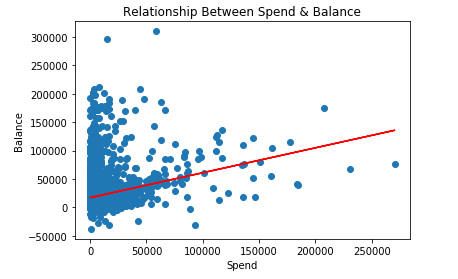### Correlation Coefficient

`cardSpendBal['Balance'].corr(cardSpendBal['Spend'])`

``0.24491708661994918``

```# Get Correlation Coefficient and P Value from scipy.stats import pearsonr pearsonr(cardSpendBal['Balance'],cardSpendBal['Spend'])```

``(0.24491708661994915, 2.7223564061743213e-43)``

## Concluding thoughts

Correlation Analysis is one of the common data analysis steps. It is a required step and is very helpful in telling a story to business stakeholders.

Tags
Most PopularJun 18, 2020Jul 23, 2020Jun 19, 2020Jun 19, 2021